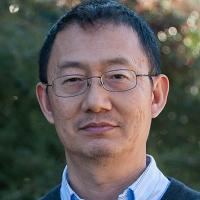The Applied Mathematics Group in the Department of Mathematics at the University of Georgia has scientific interests in Mathematical Biology, Mathematical Ecology, Computational Mathematics, Approximation Theory, Dynamical Systems, Signal Processing, Control Theory, and Mathematical Finance. The research topics its members are currently investigating include epidemiology and systems biology of malaria, stability in population dynamics models, systems ecology, network analysis, microbial community analysis, compressive sensing, video restoration, stochastic filtering, and multivariate spline methods for applications such as numerical solutions of partial differential equations, scattered data interpolation and fitting, and image analysis.

PersonnelMalcolm R. AdamsJosiah Meigs Distinguished Teaching Professor

Much of my work has been in the area of integrable Hamiltonian systems. In general I am interested in properties and applications of differential equations.Juan B. GutierrezAdjunct Associate Professor of Mathematics

I am interested in multi-scale problems in mathematical biology as described here.Cuiyu HeLimited Term Assistant Professor

My research interest lies broadly in the area of Computational and Applied mathematics, more specifically, the analysis and application of the Finite Element Methods (a numerical method for solving PDEs). Below is a list of detailed topics that I am currently interested in:

-- Adaptive mesh refinement and a Posteriori error estimation for finite element methods;

-- Unfitted finite element methods including particularly CutFEM and IFEM;

-- Shape optimization problem using unfitted FEMs;

-- Local Flux Recovery for FEMs.Ming-Jun LaiProfessor

My current research interests are 1) GBC(generalized barycentric coordinates) for polygonal splines; 2) Matrix and Data Tensor Completion; 3) Numerical Solution of Helmholtz equation and Maxwell equations; 4) Graph Clustering and Semi-supervised Learning, as well as 5) Scattered Data Fitting.Lin MuAssistant Professor

- Numerical Analysis

- Numerical Methods for Partial Differential Equations

-Scientifice ComputingQing ZhangProfessor

Applied Mathematics, Applied Probability, Stochastic Optimal Control, Nonlinear Filtering, Mathematical Finance